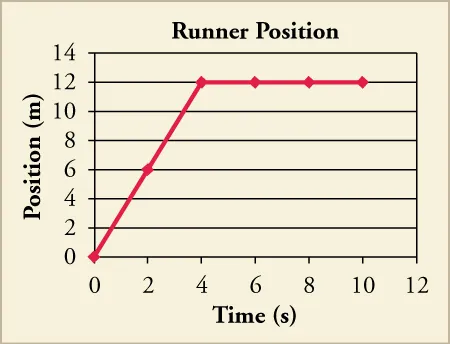Physics

# Multiple Choice

PhysicsMultiple Choice

### Multiple Choice

#### 2.1Relative Motion, Distance, and Displacement

25 .
Why should you specify a reference frame when describing motion?
1. a description of motion depends on the reference frame
2. motion appears the same in all reference frames
3. reference frames affect the motion of an object
4. you can see motion better from certain reference frames
26 .
Which of the following is true for the displacement of an object?
1. It is always equal to the distance the object moved between its initial and final positions.
2. It is both the straight line distance the object moved as well as the direction of its motion.
3. It is the direction the object moved between its initial and final positions.
4. It is the straight line distance the object moved between its initial and final positions.
27 .
If a biker rides west for 50 miles from his starting position, then turns and bikes back east 80 miles. What is his net displacement?
1. 130 miles
2. 30 miles east
3. 30 miles west
4. Cannot be determined from the information given
28 .
Suppose a train is moving along a track. Is there a single, correct reference frame from which to describe the train’s motion?
1. Yes, there is a single, correct frame of reference because motion is a relative term.
2. Yes, there is a single, correct frame of reference which is in terms of Earth’s position.
3. No, there is not a single, correct frame of reference because motion is a relative term.
4. No, there is not a single, correct frame of reference because motion is independent of frame of reference.
29.

If a space shuttle orbits Earth once, what is the shuttle’s distance traveled and displacement?

1. Distance and displacement both are zero.
2. Distance is circumference of the circular orbit while displacement is zero.
3. Distance is zero while the displacement is circumference of the circular orbit.
4. Distance and displacement both are equal to circumference of the circular orbit.

#### 2.2Speed and Velocity

30 .
Four bicyclists travel different distances and times along a straight path. Cyclist 1 travels 95m in 27s. Cyclist 2 travels 87m in 22s. Cyclist 3 travels 106m in 26s. Cyclist 4 travels 108m in 24s. Which cyclist traveled with the greatest average speed?
1. Cyclist 1
2. Cyclist 2
3. Cyclist 3
4. Cyclist 4
31.

A car travels with an average speed of 23 m/s for 82 s. Which of the following could NOT have been the car's displacement?

1. 1,700 m east
2. 2,000 m west
3. 1,600 m north
4. 1,500 m south
32 .
A bicycle camper rides from her starting point to her first campsite one day, then continues at a different speed to a second campsite the next day. If her average speed for the two days equals the average of her speeds each day, what must be true about her journey?
1. She rode the same amount of time and a different distance each day.
2. She rode for different amounts of time and distance each day.
3. She rode the same distance and the same amount of time each day.
4. She rode the same distance but different amounts of time each day.
33 .
A car is moving on a straight road at a constant speed in a single direction. Which of the following statements is true?
1. Average velocity is zero.
2. The magnitude of average velocity is equal to the average speed.
3. The magnitude of average velocity is greater than the average speed.
4. The magnitude of average velocity is less than the average speed.

#### 2.3Position vs. Time Graphs

34.

What is the slope of a straight line graph of position vs. time?

1. Velocity
2. Displacement
3. Distance
4. Acceleration
35.

Using the graph, what is the runner’s velocity from 4 to 10 s?1. –3 m/s
2. 0 m/s
3. 1.2 m/s
4. 3 m/s

#### 2.4Velocity vs. Time Graphs

36 .
A car traveling at 10 m/s is traveling at twice the velocity 10 s later. If you made a velocity graph of this motion, what information would be obtained from the area under the graph?
1. The rate of acceleration of the car.
2. The area enclosed by the car’s trip.
3. The car’s displacement during the 10 s.
4. The velocity of the car at t = 10 s.
37 .
An object is moving along a straight path with constant acceleration. A velocity vs. time graph starts at $0$ and ends at $10\,\text{m/s}$, stretching over a time-span of $15\,\text{s}$. What is the object’s net displacement?
1. $75\,\text{m}$
2. $130\,\text{m}$
3. $150\,\text{m}$
4. cannot be determined from the information given
Order a print copy

As an Amazon Associate we earn from qualifying purchases.